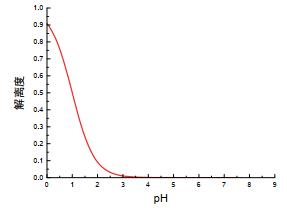## Mathematical Interpretation of Initial Curve of Acid-Base Titration between Strong Base and Weak Acid: Taking NaOH and HAc as an Example

Sun Shuzhe1,2, Zhang Zehao2,3, Zhang Bin,1

 基金资助: “基础学科拔尖学生培养计划”研究课题.  20180212Abstract

In the acid-base titration curve of titration from NaOH to HAc, shown in the sixth edition of Analytical Chemistry edited by Wuhan University, we found that there was an obvious protruding part of the curve at the initial stage of titration, which was significantly different from the curve of titration from NaOH to HCl at the same concentration. In this paper, the mathematical expression of fitting the curve is derived by using various equilibrium relations in acid-base reaction. The correlation law of acid-base titration is proved by combining with the image of mathematical function. The mathematical model can explain not only the differences between two curves, but also the reasons and influencing factors of the initial titration curve difference of weak acids by strong alkali titration.

Keywords： Analytical chemistry ; Acid-base titration ; Titration curves ; Acid-base equilibrium ; Mathematical model

Sun Shuzhe. Mathematical Interpretation of Initial Curve of Acid-Base Titration between Strong Base and Weak Acid: Taking NaOH and HAc as an Example. University Chemistry[J], 2021, 36(6): 2008008-0 doi:10.3866/PKU.DXHX202008008

### 1.1 基本公式的回顾

${K_{\rm{a}}} = [{{\rm{H}}^ + }][{\rm{A}}{{\rm{c}}^ - }]/[{\rm{HAc}}] = 1.8 \times {10^{ - 5}}$

${K_{\rm{w}}} = [{{\rm{H}}^ + }][{\rm{O}}{{\rm{H}}^ - }] = 1.0 \times {10^{ - 14}}$

$[{\rm{N}}{{\rm{a}}^ + }] + [{{\rm{H}}^ + }] = [{\rm{A}}{{\rm{c}}^ - }] + [{\rm{O}}{{\rm{H}}^ - }]$

$c = [{\rm{HAc}}] + [{\rm{A}}{{\rm{c}}^ - }]$

### 1.2 必要假设的引入

$\left[ {{\rm{HAc}}} \right] = 0.1000 - {\left[ {{\rm{Ac}}} \right]^ - }$

### 1.3 相关方程的推导

$[{\rm{N}}{{\rm{a}}^ + }] = x, [{{\rm{H}}^ + }] = y$

$[{\rm{A}}{{\rm{c}}^ - }] = x + y - \frac{{{K_{\rm{w}}}}}{y}$

$[{\rm{HAc}}] = 0.1000 - [{\rm{A}}{{\rm{c}}^ - }] = 0.1000 - x - y + \frac{{{K_{\rm{w}}}}}{y}$

$x = \frac{{0.1000{K_{\rm{a}}}}}{{{y_{\rm{a}}} + {K_{\rm{a}}}}} - y$

$y = {10^{ - {\rm{pH}}}}$

### 图1### 图2### 2.1 理论解释

#### 2.2.1 解离度与氢离子浓度的关系推导

$\begin{array}{l}{\rm{HAc ⇌ }}{{\rm{H}}^ + } + {\rm{A}}{{\rm{c}}^ - }\\{\rm{NaOH + HAc = NaAc + }}{{\rm{H}}_2}{\rm{O}}\end{array}$

$\alpha = \frac{{[{\rm{A}}{{\rm{c}}^ - }] - [{\rm{N}}{{\rm{a}}^ + }]}}{{c - [{\rm{N}}{{\rm{a}}^ + }]}} = \frac{{[{\rm{A}}{{\rm{c}}^ - }] - [{\rm{N}}{{\rm{a}}^ + }]}}{{0.1000 - [{\rm{N}}{{\rm{a}}^ + }]}}$

### 图3### 图4### 图5## 参考文献 原文顺序 文献年度倒序 文中引用次数倒序 被引期刊影响因子

Christian, G. D. Analytical Chemistry, 6th ed.; John Wiley & Sons, Inc.:New Jersey, USA, 2004; pp. 272-278.

/

 〈〉